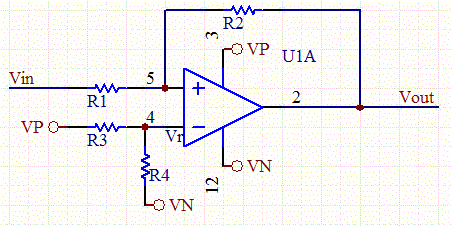# Comparator Hysteresis Calculator

This calculator will compute the resistor ratio R1/R2 and reference voltage for given high and low threshold values for a hysteresis curve, or the threshold values given the reference voltage and resistor ratio.

The most commonly know comparator is the LM339 and it's various flavors. Rail to rail op-amps can also be used as comparators. The LMV324 is one such op-amp that is affordable, and works well as a comparator.Comparator Circuit Schematic

The design equations for this calculator can be seen at:

 Input: (Note: Use minus sign "-" for negative voltages.) Positive Supply Voltage: (V) Negative Supply Voltage: (V) Results: Vth (High Threshold Voltage): (V) R2/R1 (Ratio of Resistors): Vtl (Low Threshold Voltage): (V) Vr (Reference Voltage): (V)